# Bracelet

The silver bracelet weighs 62g and contains 59g of silver. What is the purity of the bracelet?

r =  952

### Step-by-step explanation: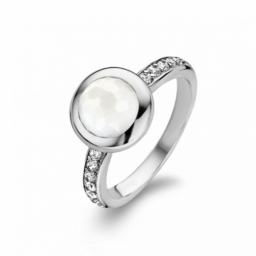Did you find an error or inaccuracy? Feel free to write us. Thank you!Tips to related online calculators
Do you want to round the number?

## Related math problems and questions:

• The gold coinThe gold coin contains 900 per mille of pure gold. The coin weighs 950g. How much per mille is gold? How many grams are gold?
• Gold coin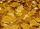Gold coin contains 964 promile pure gold, which is 7.16 g. What is the weight of coin in grams?
• Percentages 8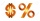What percent (%) is the amount of 12 from a base of 69? Round final result to two decimals.
• Subsets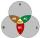How many are all subsets of set C = (97, 67, 66, 18, 59, 64)?
• Three examples per-mille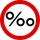A. Calculate 1.5 ‰ from 4.5 liters of blood. Express the result in milliliters. B. Calculate three ‰ from € 50,000. C. The tray they brought breakfast to the landlord's bed is made of an alloy that contains 830 ‰ of silver. How much silver did they use on
• Wood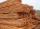Wood contains 12% water. 1 m3 of wood weighs 650 kg. How many liters of water it contains?
• Language testYour language test scores are 58, 68, 89 and 95. What is the lowest you can earn on the next test and still achieve an average of at least 79?
• ConcentrationHow many grams of 65% and 50% acid is to be mixed to give 240 g acid with a concentration of 60%?
• Sea waterMixing 62 kg of sea water with 84 kg rainwater is created water containing 3.1% salt. How many percent sea water contains salt?
• Barrel 2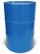Empty barrel weighs 7 kg. When it filled with water up to 45% height, weighs 60 kg. How heavy is barrel full of water?
• Alcohol mixtureFrom 55% and 80% alcohol, we have to produce 0.2 kg of 60% alcohol. How many of them do we use in the solution?
• SpiritFrom 55% and 80% spirit we would like to produce 0.2 kg of 60% spirit. How many of them we must use in a solution?
• Uranium ore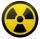Uranium ore contains 0.2% of pure uranium and pure uranium contains 0.07% of fissile uranium. How many grams of fissile uranium contains 2800 ton of uranium ore?
• Sixty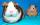Sixty percent of one-fifteen of the total is equal to thirty. What are two percent of the total?
• Percentages52 is what percent of 93?
• A semi-circular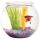A semi-circular fishbowl is filled with water and has a diameter of 10 feet. What is the total weigh of water to the nearest pound in the tank, if water weighs 62.5 pounds per cubic foot?
• Alcohol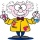6l 48% alcohol, how much 52% alcohol must be added to give 50% alcohol?